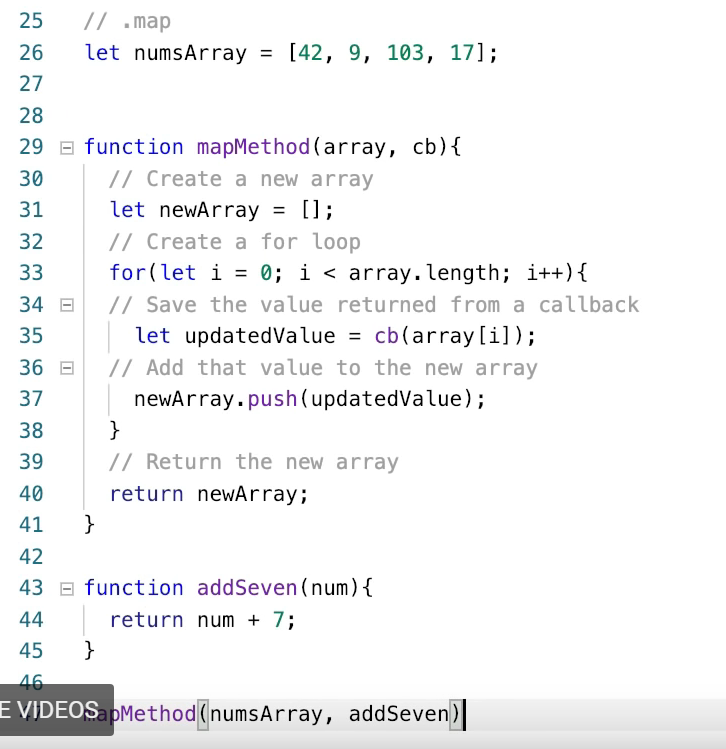JS Assignment 16: Using Callbacks in Array Methods

function exerciseOne(names){
// Exercise One: In this exercise you will be given and array called names.
// Using the forEach method and a callback as it's only argument, console log
// each of the names.
}

function exerciseTwo(cents){
// Exercise Two: In this exercise you will be given an array called 'cents'
// This array is a list of prices, but everything is in cents instead of dollars.
// Using the map method, divide every value by 100 and save it as a new array 'dollars'

return dollars;
}

This is what I have:

function exerciseOne(names){
// Exercise One: In this exercise you will be given and array called names.
// Using the forEach method and a callback as it's only argument, console log
// each of the names.
console.log(names);
}

function eachMethod(array, cb){
for(let i = 0; i < array.length; i++){
cb(array[i]);
}
}
console.log(names.forEach(exerciseOne));

function exerciseTwo(cents){
// Exercise Two: In this exercise you will be given an array called 'cents'
// This array is a list of prices, but everything is in cents instead of dollars.
// Using the map method, divide every value by 100 and save it as a new array 'dollars'
return cents / 100;
}
dollars.map(exerciseTwo);
return dollars;
}

What did I do wrong?

You are viewing a single comment. View All

### Exercise One:

It ask you to console.log each individual element, not the entire array:

``````function exerciseOne(names) {
names.forEach(elem => console.log(elem));
}``````

### Exercise Two:

You are doing code that makes no sense. You have to do this:

``````function exerciseTwo(cents) {
var dollars = cents.map(elem => elem / 100);
return dollars;
}``````

If this answers your question, please check the checkmark on the left side of this message. Thanks and Good Luck!

maximussallam (5)

malvoliothegood (365)

maximussallam (2)'s questions could be homework questions so it is best not to give him/her the answers, rather point them in the right direction or give them a similar example.

patrickvmederos (1)

@vedprad1 in the youtube video and material we are given before performing this assignment it does not make any mention of using "elem =>". My question would be how would we have known to use this => to get the correct answer? Did I miss something?edit: after messing around for with it for awhile, it tells me that this is a correct answer:

function exerciseOne(names){
// Exercise One: In this exercise you will be given an array called 'names'.
// Using the forEach method and a callback as it's only argument, console log
// each of the names.

names.forEach(function (names){
console.log(names);
}

)}